Courses

# Test: Highway

## 25 Questions MCQ Test GATE Civil Engineering (CE) 2022 Mock Test Series | Test: Highway

Description
This mock test of Test: Highway for GATE helps you for every GATE entrance exam. This contains 25 Multiple Choice Questions for GATE Test: Highway (mcq) to study with solutions a complete question bank. The solved questions answers in this Test: Highway quiz give you a good mix of easy questions and tough questions. GATE students definitely take this Test: Highway exercise for a better result in the exam. You can find other Test: Highway extra questions, long questions & short questions for GATE on EduRev as well by searching above.
QUESTION: 1

### The speed of overtaking and overtaken vehicles are 90 Kmph and 72 Kmph respectively. Calculate the overtaking sight distance. Assume acceleration of overtaking vehicle as 0.8 m/s2 and traffic stream moves in one way.

Solution: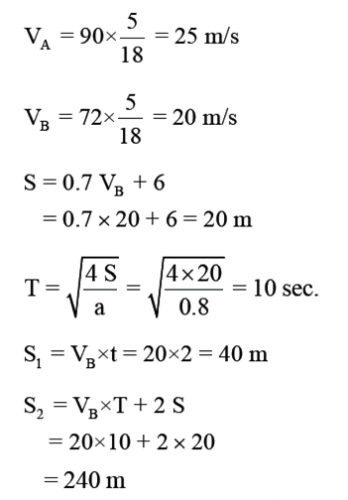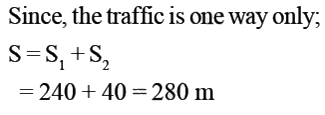QUESTION: 2

### A car moves at a speed of 90 Kmph on a road having 5% downward gradient. If the reaction time of the driver is 2 seconds, assuming that f = 0.15, calculate the distance moved by the vehicle before the car stops finally after the brakes are applied ? Take g = 10 m/s2

Solution:

Distance moved by the vehicle before the car stops finally after the brakes are applied = Braking Distance.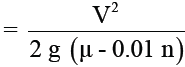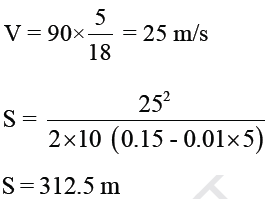QUESTION: 3

### A roundabout is provided with an average entry width of 8.4 m, width of weaving section as 14 m, and length of the weaving section between channelizing islands as 35 m. The crossing traffic and total traffic on the weaving section are 1000 and 2000 PCU per hour respectively. The nearest rounded capacity of the roundabout (in PCU per hour) is

Solution: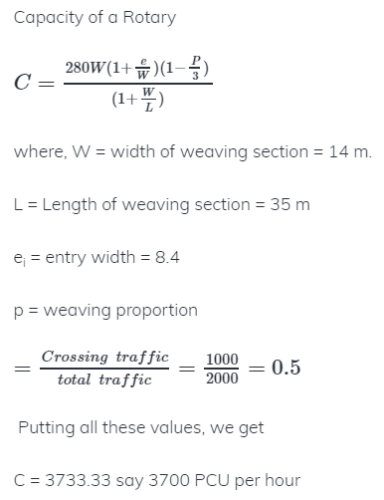QUESTION: 4

The traffic count on road is proposed to be 2500 commercial vehicles per day. Growth of traffic is 5 percent vehicle damage factor = 2.5. The design Life of pavement is 10 years and traffic distribution factor can be adopted as 0.5. What are the cumulative standard axles (MSA)?

Solution: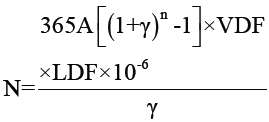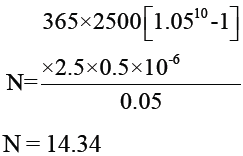QUESTION: 5

In cement concrete pavement, tie bars are installed in:

Solution:

Dowel Bars are provided at expansion joint/contraction (transverse joint) and is designed for bearing, bending and shearing stress.
Tie bars are provided at longitudinal joint and they are designed for warping stress and holding slab in position.

QUESTION: 6

If the modulus of sub-grade reaction of standard plate of 30 cm diameter is 20 Kg/cm3, the value for a standard plate of 75cm diameter is:

Solution: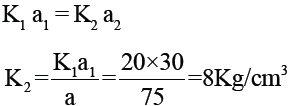QUESTION: 7

The bitumen content in the Marshal specimen of bitumenous concrete is 5% of the total weight of the mix.
The theoretical and measured unit weights of the mix are 2.5 g/cm3 and 2.4 g/cm3 respectively. The bitumen has specific gravity of 1. The percentage voids in mineral aggregates filled with bitumen (VFB) are:

Solution: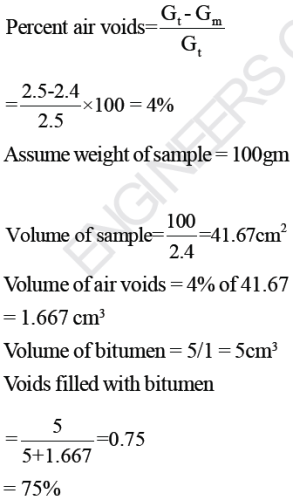QUESTION: 8

The consistency and flow resistance of bitumen can be determined from which of the following?

Solution:

Ductility Test- Distance in cm to which a standard briquette of size 10mm x 10mm can be stretched before the thread breaks at a standard temperature.
Penetration Test- Represents hardness/softness of bitumen by measuring the depth of penetration 1/10 of mm to which a standard needle carrying a weight of 100 gram penetrates in 5 seconds.
Softening point Test- The temperature at which a standard ball passes through the sample of bitumen and falls when heated under water or glycerine. It represents the fluidity of binder.
Viscosity Test- It is the time taken in seconds by 50ml of material to flow through the container to a specified orifice of size 10mm under standard conditions and temperature.

QUESTION: 9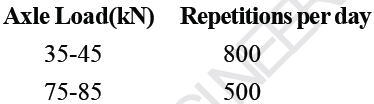The standard axle Load is 80kN. Equivalent daily number of repetitions for the standard axle Load are:

Solution: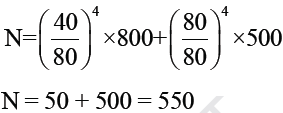QUESTION: 10

Radius of relative stiffness of cement concrete pavement does not depend upon which one of the following?

Solution: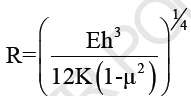QUESTION: 11

What will be the ruling radius of a horizontal curve on a national highway for a design vehicle speed of 100 Km/h assuming allowable super elevation to be 7% and Lateral friction as 0.13?

Solution: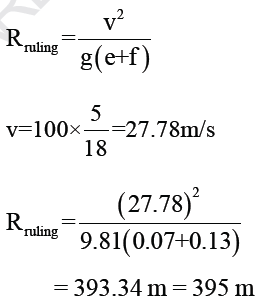QUESTION: 12

For a given road, safe stopping sight distance is 80m and passing sight distance is 300m. What is the intermediate sight distance?

Solution:

ISD = 2 × SSD = 2 × 80 = 160m

QUESTION: 13

A summit curve is formed at the intersection of a 3% upgrade and a 5% downgrade. What is the length of the summit curve in order to provide a stopping distance of 128m?

Solution: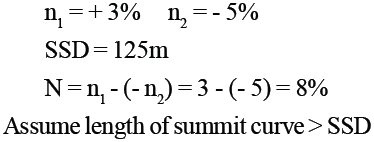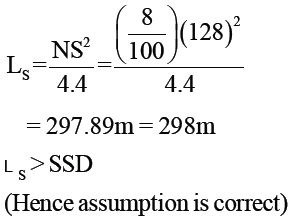QUESTION: 14

On a road the free speed was 65 Kmph and the space headway at jam density was 6.25m. What is the maximum flow which should be expected on this road?

Solution: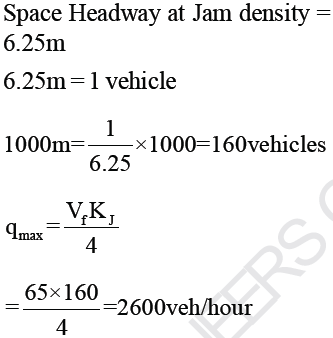QUESTION: 15

In spot speed study, which one is considered the design speed on highway?

Solution:

In cumulative speed distribution following values must be designed for:
98th percentile speed: check for design adequacy= design speed for highway
85th percentile speed: Upper speed limit for vehicles
15th percentile speed: Lower speed limit for vehicles

QUESTION: 16

In floating car method, following observations are made when floating car moves in N-S direction

No. of Vehicles overtaken by the test vehicle = 155

No. of vehicles overtaking the test vehicle = 105

Vehicles counted in S-N direction when test vehicle moves in N-S direction =80

Vehicles counted in N-S direction when test vehicle moves in N-S direction = 75

Length of road stretch = 0.5 Km

Speed of test vehicle = 20 Kmph

Calculate the average journey time of the traffic stream in N-S direction?

Solution: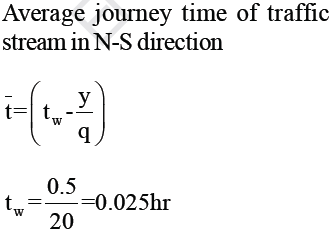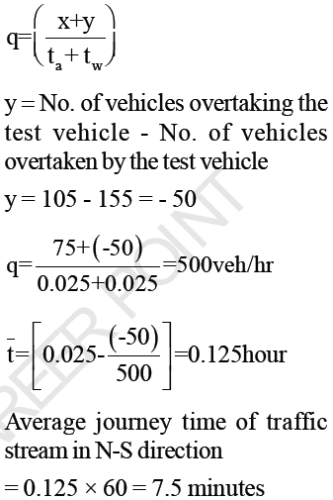QUESTION: 17

A district road with a bituminous pavement has a horizontal curve of 1000 m for a design speed of 75 km ph. The super-elevation is

Solution: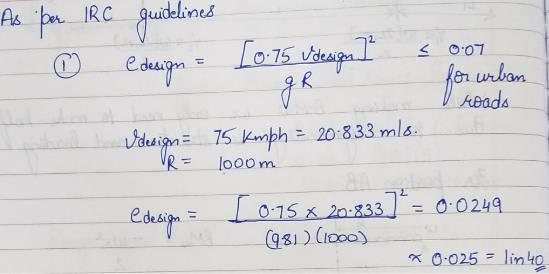QUESTION: 18

A mixture contains coarse aggregates (GS = 2.7), fine aggregates (GS = 2.9), Mineral filler (GS = 1.5) in the proportion 50:40:10 (by weight). These materials are mixed with bitumen (GS = 1.2) and compacted, the unit
weight becomes 2300 Kg/m3 and contains 5% voids. Calculate the voids in mineral aggregates for the given specimen?

Solution: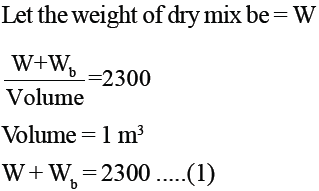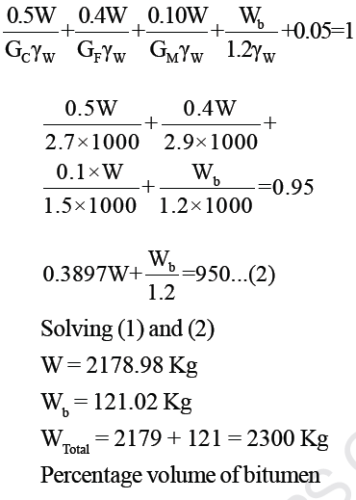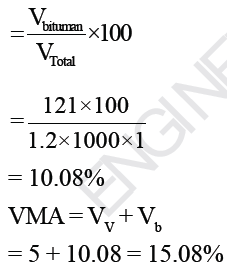QUESTION: 19

A two Lane urban road with one way traffic has a maximum capacity of 2000 vehicles/hour. Under the jam condition the average length occupied by the vehicles is 5m. The speed versus density relationship is
linear. For a traffic volume of 1200 vehicles per hour, the density (in vehicles/Km) is:

Solution:

Under Jam condition average spacing between vehicles = 5m

Number of vehicles per Lane per Km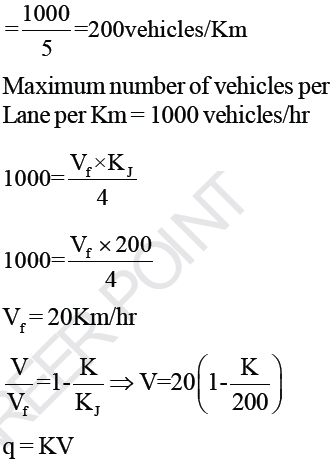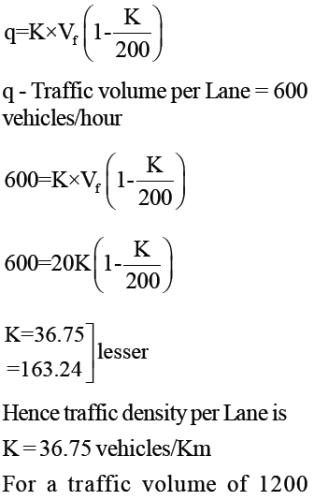vehicles/Km

traffic density is 73.5 vehicles/Km

*Answer can only contain numeric values
QUESTION: 20

An observer counts 300 vehicles/hour at a specific highway location. Assume that the vehicle arrival at the Location is Poisson’s distribution, the probability of having one vehicle arriving over a 30 second time interval is.......

Solution:

Average number of vehicles in 30 seconds interval: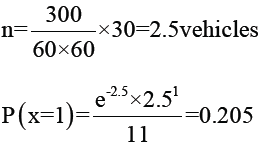QUESTION: 21

On a specific highway, the speed density relationship follows the Greenberg’s Model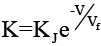, where vf and KJ are the free flow speed and jam density respectively when the highway is operating at capacity, the density obtained as per this model is:

Solution: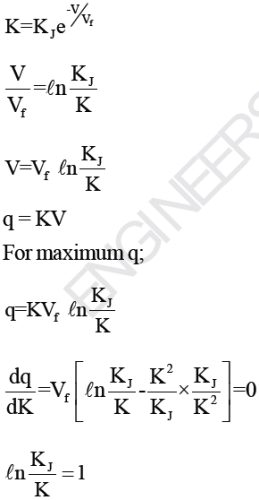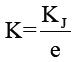QUESTION: 22

The shape of the STOP sign according to IRC: 67-2001 is:

Solution:

Stop sign is an example of a regulatory sign . Although regulatory signs are circular in shapes but stop sign is an exception having octagonal shape.

QUESTION: 23

If the pressure carried by a CBR specimen at 2.5mm penetration is 3.5 N/mm2, the CBR of the soil is:

Solution: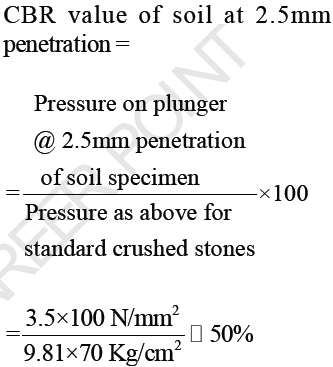*Answer can only contain numeric values
QUESTION: 24

The width of expansion joint gap is 2.5cm, in a cement concrete pavement. If the minimum and maximum temperature of slab are 10oC and 30oC, calculate the spacing between expansion joints. Assume coefficient of thermal expansion of concrete is 10 × 10-6 per oC. Assume that joint filler can be compressed up to 50%.........m

(Important - Enter only the numerical value in the answer)

Solution: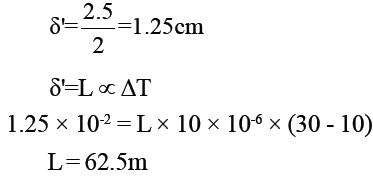QUESTION: 25

If the load, warping and frictional stresses in a cement concrete slab are 210 N/mm2, 290 N/mm2 and 10 N/mm2 respectively, the critical combination of stresses during summer mid-day is:

Solution:

During summer mid-day, the critical combination of stresses
= 210 + 290 - 10 = 490 N/mm2